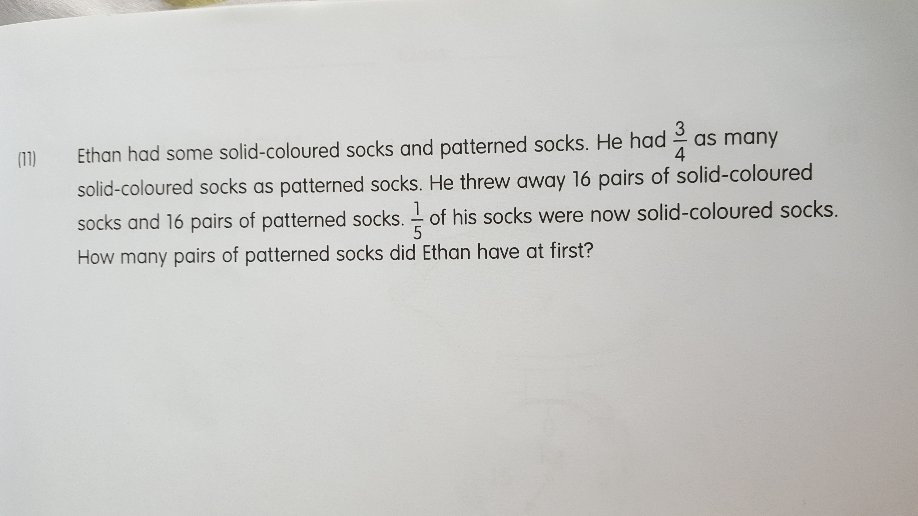# QuestionLet the original total number of socks be 7X

Let the total number of socks left be 5Y

3X – 16 = Y

4X – 16 = 4Y

4Y = 4(3X-16) = 12X – 64

4X – 16 = 12 X – 64

12X – 4X = 64-16

8X = 48

X=6

Original number of patterned socks = 4X = 24

Test for correct answer

18 – 16 = 2

24 – 16 = 8

Total number of socks left is 10. Since 2 = 1/5 of 10, the answer is proven to be correct.

0 Replies 1 Like ✔Accepted Answer